# Optimization using maximum network flow

In this article, I look at the use of maximum network flow algorithms and how they can be used to find optimal solutions to practical problems in distribution, transportation, finance and many other industries.

# What is an optimization problem?

We all have an intuitive understanding of what optimization means. It’s about finding the ‘best’ solution to a problem. Maximum network flow is a single-objective optimization algorithm. This means that it can only be used where we have a single objective against which to optimize. So, we need to decide in what respect our solution should be the best. Do we want to find the cheapest journey or the fastest? Do we want to maximise our potential profit or minimize our potential losses? We have to choose a single objective.

To meet the mathematical requirements for optimization, we also need to express our objective as a function of decision variables. If we want to optimize the flow of passengers on a rail network, for example, we need to know passenger volumes at each station and how many of them want to reach each destination. Our goal is then to maximise the number of passengers getting to their desired destination.

# What kinds of problem can be solved with maximum network flow?

Network flow uses a particular type of graph structure illustrated in the diagrams below. If we can represent a problem in this way then we can use maximum flow to find an optimal solution. It sometimes requires a bit of lateral thinking but this is possible with a broad range of real-world problems.

## Transportation and Distribution Networks

The network flow problem was first formulated as a model of railway traffic. The objective was to maximise the flow of passengers between cities. Equivalent transportation problems can be solved with network flow by simply substituting, for example, cities with ports and passengers with shipping containers.

## Meeting Demand from Storage

Problems where stores of something are used to meet demand can be represented as a network flow. In a water network, for example, we have water reservoirs and water consumers (these could be cities, streets, buildings or individual machines depending on the scale of our modelling). Network flow can find an optimal flow of water from supplying nodes (i.e. reservoirs) to the consuming nodes on the network. We can take the same approach with an electrical circuit, with components such as batteries being sources of electrical current and components such as lights, motors and microchips being the consumers. The approach could work equally well with customers waiting for products to be despatched from warehouses or hospitals and patient waiting lists.

## Financial Modelling

In some cases, we can treat financial transactions as flows. Payments by customers are flows into bank accounts (which are stores of money). Payments we make to suppliers are flows out of bank accounts. Loans and invoices can be considered “consumers” of money.

It is possible to stretch the analogy even further and use network flow to optimize financial netting. Say we have a financial market where lots of traders are trading with one another. Instead of executing every financial transaction, we can use maximum network flow to calculate net financial positions, which can be settled with single transactions between parties. Netting can also be used where, for example, companies make many purchases and sales to one another. In this case, we net each company’s sales and purchase invoices to find the net balance between each pair of companies.

# How does maximum network flow work?

To illustrate how to use the algorithm, we’ll work with a simple example of a fictional place with three water reservoirs serving five cities. As shown in table 1, the capacities of the reservoirs exactly match total demand across all cities. This is not ideal in terms of resilience to varying demand but there is just enough water to go around.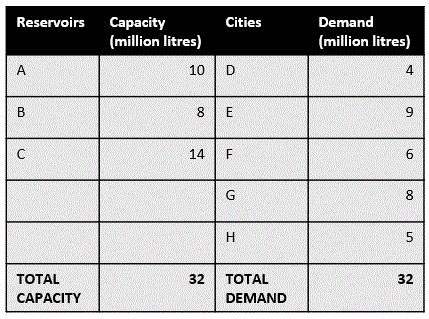Table 1. Supply nodes (reservoirs) and consuming nodes (cities) in our simple example

We also know which reservoirs have pipelines connecting them to each city. This is given to us in figure 1.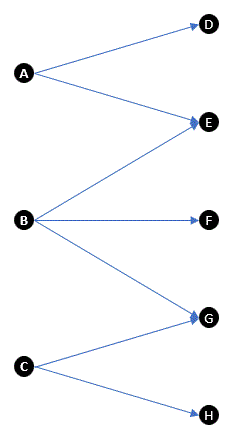Figure 1. Pipelines connecting reservoirs (A to C) to cities (D to H)

To use network flow, we need create a network between two points that enables us to optimize against our objective (in this case, satisfy the total water demand across all cities). There are no points in Figure 1 that represent demand across all cities or all available supply. We can overcome this problem by creating two artificial points, connecting one to all supplying reservoirs and one to all cities. We then put the capacities of the reservoirs and the water demands of the cities on these new connections. This gives a network like that in Figure 2. This structure might seem a little odd but the reasons will soon become clear.

Each circle in figure 2 is called a vertex and each arrow is called an edge. Vertex S is the source vertex and vertex T is the sink or target vertex. Our algorithm will maximize the flow from source (S) to target (T) by pushing flow (water in our example) along the edges. The algorithm can push flow forward (e.g. from A to D) or backward (from D to A) along the edges. Where edges have a capacity (i.e. the S-connected and T-connected edges), the flow is not permitted to exceed this limit.

The first step in the algorithm is simply to push the total value of each edge leaving S to the connected vertices as shown in figure 3. We can think of this as the rain filling our reservoirs or, in a different example, charging the batteries on an electrical circuit. We do this because network flow uses the concept of excess. For each of the real vertices (A to H), we monitor the total inbound flow and the total outbound flow. The excess is the inbound flow minus the outbound flow. When we push the maximum possible amount from S across each connected edge, we maximise the excess at each of supplying vertices (A to C). The goal of the algorithm is to eliminate these excesses by pushing flow to other connected vertices.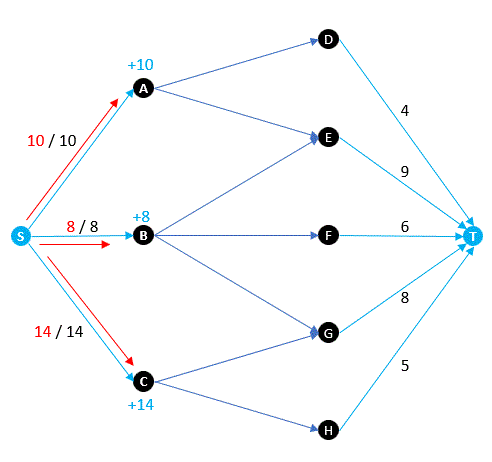Figure 3. As shown in red, the first step in a network flow is to push from the source vertex (S) to all adjacent vertices creating excess at these vertices (shown in blue)

Researchers have developed numerous ways of pushing flow around a network, varying in terms of their best, average and worst case efficiency (see references below). Figure 4 illustrates how the forward and backward pushing of flow around the network begins to eliminate excesses at the vertices. Essentially, the algorithm tries to push as much flow as possible from S to T. If it is not possible to push from a vertex towards T because the capacities of all outbound vertices have been reached, then the excess flows from that vertex back towards S. This continues until the excesses at all vertices except S and T have reached zero.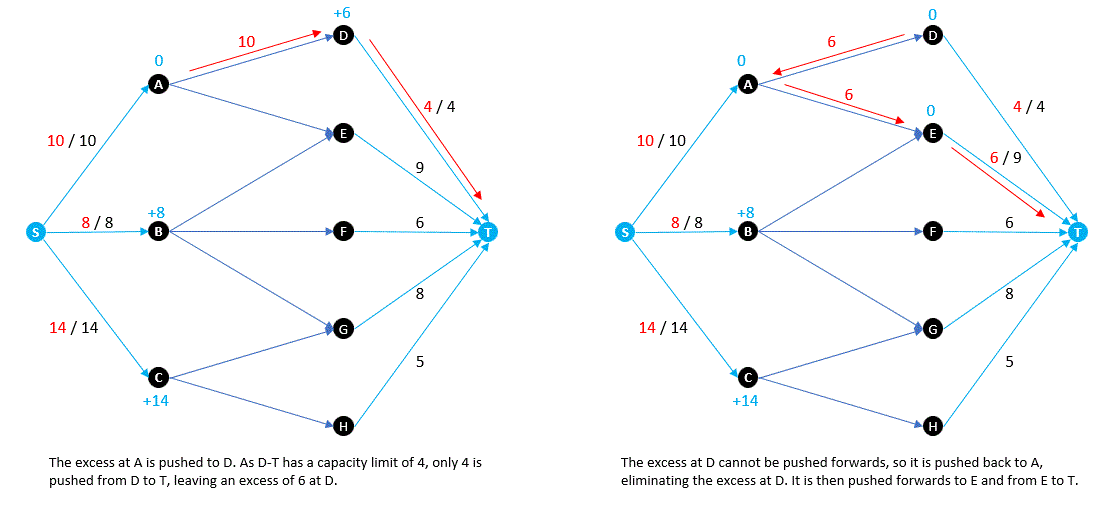Figure 4. Starting with each S-connected node having a maximum excess, each iteration of the algorithm pushes flow around the network to reduce excess across all vertices

Figure 5 shows a maximum flow for our simple example. The inflows and outflows at each vertex (except S and T) are equal, so all excesses have reached zero and the algorithm terminates. We can see that there is some unused capacity at reservoir C and some unmet demand at city F. Given the current set of edges (i.e. pipelines between reservoirs and cities), it is not possible to do any better. From a practical perspective, the maximum flow result offers some valuable insights. We now know that the optimal result is a flow of 31 million litres of water against a demand of 32 million litres (i.e. we meet almost 97% of total demand). We can also see that we satisfy 100% of demand at D, E, G and H but city F only receives 5 out of 6 million litres (or 83%) of its requirement. Looking at the left of the graph, we see that reservoirs A and B supply at 100% of their capacity and around 93% of reservoir C’s capacity is used. If we were city planners, we can see that a pipeline from C to F makes it possible to satisfy all demand by using 100% of available supply. We can also tell that cities D, E or F are only connected to fully utilized reservoirs with no spare capacity, so any increase in their demand cannot be satisfied.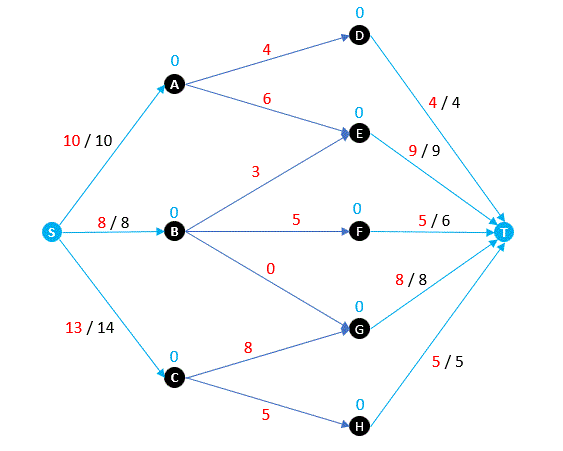Figure 5. A maximum flow result for the example reservoirs and cities problem

# Maximum network flow in practice

I’ve used maximum network flow to solve financial problems for several years. It has provided results in cases with tens of thousands of vertices and millions of edges, though there can be memory and run-time challenges to solving very large problems. The fact that the algorithm guarantees an optimal result without breaking any constraints is valuable in these environments where constraints are often legal or regulatory. I’ve also modified the algorithm to cope with global constraints and other requirements. In our example, a global constraint might arise if the reservoirs and cities were spread across multiple states and these states limited the total transfer of water out of their state. Another non-standard constraint might be that we must have some form of prioritization. In the example, we might be required to use all of the flow from certain reservoirs before we are allowed to start using water from others. Such requirements can also be met with minor modifications to the algorithm.

# How do I find out more about network flow algorithms?

The first solution to the maximum flow problem was the Ford-Fulkerson algorithm published in 1956. Since then, faster variants have been published by numerous authors. A list of algorithms and their computational performance can be found at Maximum flow problem — Wikipedia.

A book exclusively about network flow algorithms written by David Williamson can be found at Network Flow Algorithms (networkflowalgs.com).

--

--